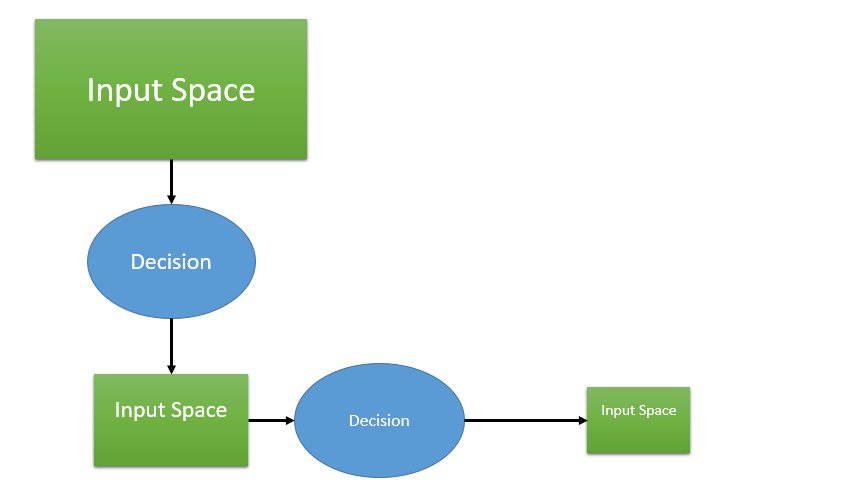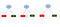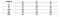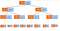# Recursion Introduction and Identification

Recursion is one of the fundamental concept of computer science and it is also one the confusing and toughest topic in computer science. Only way to understand recursion is to understand recursion. Yeah in the above line I already gave you the definition of recursion. If you ask anyone what is recursion they probably going to say a function calling itself. In text book definition:

“Recursion is the process of defining a problem (or the solution to a problem) in terms of (a simpler version of) itself.”

Recursion works like magic. In this series of tutorial I will try my best to uncover the mystery of the beautiful world of recursion. Do not get me wrong, I am not a Jedi of recursion. Back in my undergraduate days it is one of the topic that I fear most. So I am trying my best to overcome the fear of recursion by learning it in the proper way. And you know what they say: “Best way to learn a concept by teaching it.” Recursion is not necessary for itself, various data structure and programming concept are highly depended upon on recursion for example:

1. Tree data structure.

2. Graph

3. Dynamic programming.

4. Backtracking.

5. Divide and conquer.

So by this now you may have convinced that how important it is. Let’s start our recursive journey.

So the flow of this tutorial will be the following steps:

1. Make Input/output smaller.

2. Decision space

3. Recursion Tree

Make Input/output (I/O) smaller: Do me favor, please Google “recursion tutorial”. You will see every tutorials out there telling you that recursion is nothing but a function calling itself on smaller input. They are 1000% right. But no one is telling you that why we are calling on smaller input and how the input become smaller?

In recursion we are not making input smaller, input gets smaller automatically based on some decision we take. So our primary goal is not make input smaller but to take some decision, based on that decision our input will become smaller automatically.Making small the input space

2. Decision space:

There is a formula:

Recursion= Choice + Decision

In every recursion problem, there must be some sort of choice involve, based on that choice we have to take a decision. Based on that decision we are actually making our input smaller. So decision space is nothing but a bunch of choices we have in a particular scenario. This concept can be more understandable once we start working with examples.

3. Recursive tree:

This is the most import aspect of recursion. For any problem if are able to generate a recursion tree for that problem, then writing code will be a piece of cake. For any recursion problem if I am able to draw the recursive tree, I consider that the problem is solved. In this section we will look into an example. The example we are using is “power set”.

For example we are given a string “ABC”, we have to generate all the possible subsets possible. As there are three(3) letters, so there are 2³(8) possible subset possible: “”,”a”,”b”,”c”,”ab”,”ac”,”bc”,”abc”.

Now question arises given that question, how can we say that it is a recursion question? Let’s dig deep into this question.Choices and Decision

For generating power set, for every letter from the input we have a choice whether to take this input or not. As I told earlier every recursion problem has some sort of choice, and based on those choice we took some decision. In this problem we have choices to take an item into consideration or not. As this problem includes both choices and decision so it is recursion problem. Lets look at the below tableSubset generation

From this table we can see the details of the choice and decision space and based on our decision which subset is generated. Now lets see how we are going to generate recursive tree:Recursive tree

With the help of above recursion tree we will try to understand how subsets are generated. Let’s consider the blue rectangle as our input and orange rectangle as our output.

1. At first the output filed is empty.

2. Then we have to take a decision whether to take “A” or not. Based on our decision we will have two branches. Two branches mean two output. That is why in the next layer we have two output in one of them we are considering “A” and in another one we did not consider. A. Also if you look closer our input become small as we are not considering “A” anymore so we can discard “A” from our input.

3. In the next layer we will take similar decision for “B” and “C”.

4. If we look closer in the leaf node we can see in the leaf node input size is 0, and we get our desired subset as the output. This is the most important observation, in recursion we always have the desired output in the leaf node of the recursion tree.

So in this short tutorial we see discuss, what is recursion, how it works and we also see an example. So for every recursion problem: we have to make input smaller based on some choices and decision. After finding out what choice and decision to make we have to generate the recursion tree.

Then what????

Then nothing. You solved the problem already. Now writing code is piece of cake. In the next tutorial we will see the python implementation of this problem.

Interested in building game changing products.